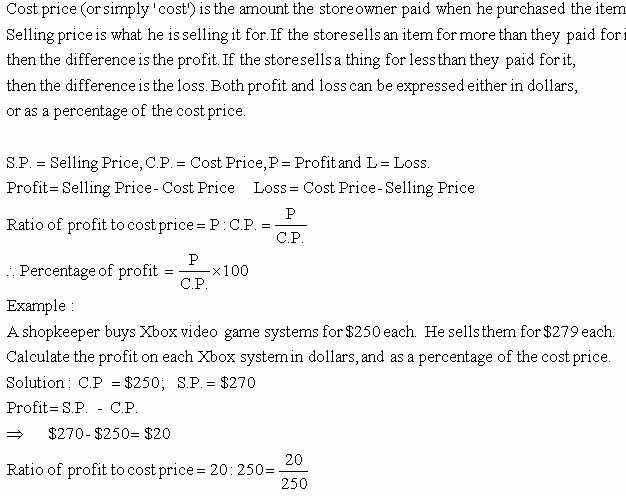Email us to get an instant 20% discount on highly effective K-12 Math & English kwizNET Programs!

#### Online Quiz (WorksheetABCD)

Questions Per Quiz = 2 4 6 8 10

### MEAP Preparation - Grade 7 Mathematics1.111 Finding Profit PercentagePercentage of profit = (20/250) x 100 = 0.08 = 8% Directions: Answer the following questions. Also write at least ten examples of your own.
 Q 1: Cost price = \$6500 and selling price = \$9000, what is the profit percentage?6 13/38%38 6/13%13 6/38% Q 2: Cost price = \$290 and selling price = \$300, what is the profit percentage?13 3/29%29 3/13%3 13/29% Q 3: Cost price = \$3000 and selling price = \$3300, what is the profit percentage?30%10%15% Q 4: Cost price = \$5000 and selling price = \$6000, what is the profit percentage?15%30%20% Q 5: Cost price = \$3500 and selling price = \$3700, what is the profit percentage?6 5/7%5 5/7%7 5/7% Q 6: Cost price = \$6000 and selling price = \$8000, what is the profit percentage?33 1/3%1 3/33%5 1/33% Q 7: Cost price = \$3600 and selling price = \$4000, what is the profit percentage?9 1/11%11 1/9%1 9/11% Q 8: Cost price = \$2500 and selling price = \$3000, what is the profit percentage?20%15%25% Question 9: This question is available to subscribers only! Question 10: This question is available to subscribers only!